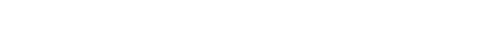glMapGrid.3glOpenGL man pages

```

```

## Name

```  glMapGrid1d, glMapGrid1f, glMapGrid2d, glMapGrid2f - define a	one- or	two-
dimensional mesh

```

## C Specification

```  void glMapGrid1d( GLint un,
GLdouble u1,
GLdouble u2	)
void glMapGrid1f( GLint un,
GLfloat u1,
GLfloat u2 )
void glMapGrid2d( GLint un,
GLdouble u1,
GLdouble u2,
GLint vn,
GLdouble v1,
GLdouble v2	)
void glMapGrid2f( GLint un,
GLfloat u1,
GLfloat u2,
GLint vn,
GLfloat v1,
GLfloat v2 )

```

## Parameters

```
un  Specifies	the number of partitions in the	grid range interval [u1, u2].
Must be positive.

u1, u2
Specify the mappings for integer grid domain values i=0 and i=un.

vn  Specifies	the number of partitions in the	grid range interval [v1, v2]
(glMapGrid2 only).

v1, v2
Specify the mappings for integer grid domain values j=0 and j=vn
(glMapGrid2 only).

```

## Description

```  glMapGrid and	glEvalMesh are used in tandem to efficiently generate and
evaluate a series of evenly spaced map domain	values.	 glEvalMesh steps
through the integer domain of	a one- or two-dimensional grid,	whose range
is the domain	of the evaluation maps specified by glMap1 and glMap2.

glMapGrid1 and glMapGrid2 specify the	linear grid mappings between the i
(or i	and j) integer grid coordinates, to the	u (or u	and v) floating-point
evaluation map coordinates.  See glMap1 and glMap2 for details of how	u and
v coordinates	are evaluated.

glMapGrid1 specifies a single	linear mapping such that integer grid
coordinate 0 maps exactly to u1, and integer grid coordinate un maps
exactly to u2.  All other integer grid coordinates i are mapped such that

u = i(u2-u1)/un + u1

glMapGrid2 specifies two such	linear mappings.  One maps integer grid
coordinate i=0 exactly to u1,	and integer grid coordinate i=un exactly to
u2.  The other maps integer grid coordinate j=0 exactly to v1, and integer
grid coordinate j=vn exactly to v2.  Other integer grid coordinates i	and j
are mapped such that

u = i(u2-u1)/un + u1

v = j(v2-v1)/vn + v1

The mappings specified by glMapGrid are used identically by glEvalMesh and
glEvalPoint.

```

## Errors

```  GL_INVALID_VALUE is generated	if either un or	vn is not positive.

GL_INVALID_OPERATION is generated if glMapGrid is called between a call to
glBegin and the corresponding	call to	glEnd.

```

## Associated Gets

```  glGet	with argument GL_MAP1_GRID_DOMAIN
glGet	with argument GL_MAP2_GRID_DOMAIN
glGet	with argument GL_MAP1_GRID_SEGMENTS
glGet	with argument GL_MAP2_GRID_SEGMENTS

```

```  glEvalCoord, glEvalMesh, glEvalPoint,	glMap1,	glMap2